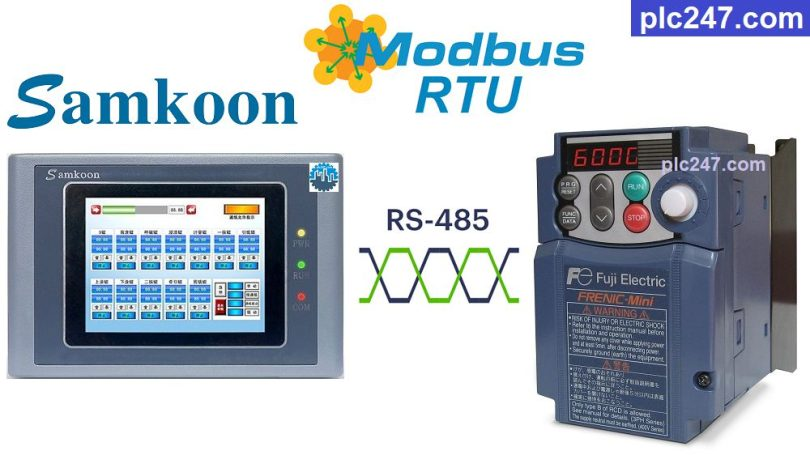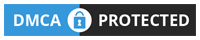# Samkoon HMI “Modbus RTU” Fuji Frenic TutorialWritten by

Hello friends!
Fuji Frenic inverter is a commonly used inverter on the market and it originated in Japan, today plc247.com will guide you to use Samkoon HMI to control it through Modbus-RTU protocol.

In this project I use Fuji Frenic Mini (Similar Multi, Mega, Eco models) and HMI Samkoon SK-070HS.

#### Samkoon HMI & Fuji Frenic VFD “Wiring Diagram”

Diagram of RS485 communication between HMI Samkoon and FUJI Frenic Mini

#### Fuji Frenic “Modbus RTU” Parameters Setting

We need to set the following communication parameters so that the Fuji Frenic inverter can be controlled via Modbus-RTU protocol:

• H30 = 3 (VFD Control via RS485 Port)
• Y01 = 1 (Slave Address)
• Y02 = 0
• Y03 = 2
• Y04 = 2 (9600bps)
• Y05 = 0 (Data: 8bit)
• Y06 = 1 (Parity Check: Even)
• Y07 = 1 (Stop Bit: 1)
• Y10 = 0 (Modbus-RTU Selection)
• Y99 = 0
##### Fuji Frenic Modbus Registers Address

+ Frequency Reference Address = S(07H) + 05 = 705 (hexa) = 1797 (dec)

+ Operation Command Address = S(07H) + 06 = 706 (hexa) = 1798 (dec)

• Set Word “1798” = 1 >>> Forward Run
= 2 >>> Reverse Run
= 0 >>> Stop Run

+ Output Frequency Address = M(08H) + 09 = 809 (hexa) = 2057 (dec)

+ Output Current Address = M(08H) + 11 = 80B (hexa) = 2059 (dec)

+ Output Voltage Address = M(08H) + 12 = 80C (hexa) = 2060 (dec)

#### Samkoon HMI Modbus Configuration

In order for the Samkoon HMI to control the Fuji Frenic inverter, we need to set the Modbus communication parameters same between the two devices

### HMI Programming

+ Interface Panel

+ Setting Frequency

+ Run Forward

+ Run Reverse

+ Stop Command

+ Frequency Output

+ Current Output

+ Voltage Output

### Project Video Tutorial

======

##### Reference